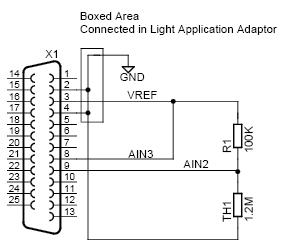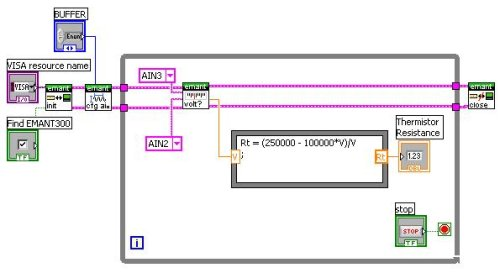The following information is for the EMANT300, EMANT380

# Measure a medical grade 1.2M @ 25c thermistor

Question: I would like to use the EMANT300 USB DAQ to measure a medical grade thermistor which has a resistance @ 25c of around 1.2M. I know that I will need to reduce the current excitation level to below 39uA, what would be the best way to do this?Answer: Connect the thermistor to the 2.5V voltage reference through a 100K 1% resistor, the voltage (V) across the 100K resistor is

V = 2.5*100000/ (Rth+100000)

where Rth is the resistance of the thermistor in ohms.

Moving the equation around gives

Rth = (250000 - 100000V)/V

Enable the input buffer so that the analog input impedance does not load your circuit. Requires the latest development language driver. Download driver and example program from info.emant.com (for EMANT customers only)

LabVIEW DiagramC# code

Emant300 DAQ = new Emant300();
DAQ.Open();
DAQ.ConfigAnalog(2.5, Emant300.POLARITY.Unipolar, 100, Emant300.VREF.V2_5, true);
volt = DAQ.ReadAnalog(Emant300.AIN.AIN3, Emant300.AIN.AIN2);
R = (250000 - 100000 * volt) / volt;
Console.WriteLine(R);
DAQ.Close();